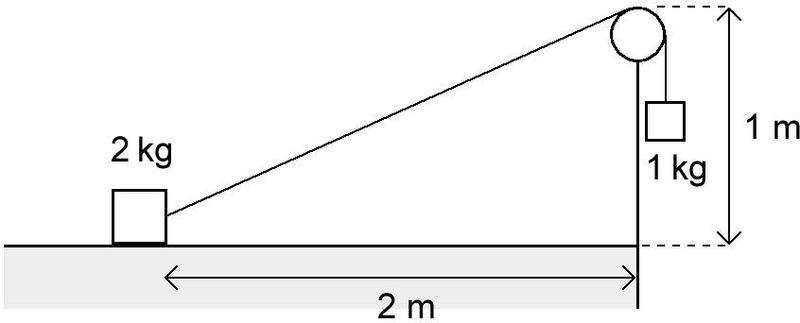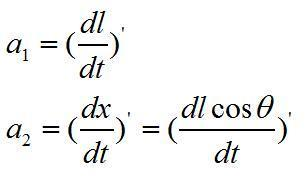# Difficult mechanics problem

zxfhero
1. A mass of 2 kg is held at rest on a rough horizontal table with coefficient of friction μ = 0.5. A string is attached to the mass and is hung over a smooth pulley. A 1 kg mass is suspended from the other end of the string. The top of the pulley is at a height of 1 m above the table surface, and the 2 kg mass is initially at a horizontal distance of 2 m away from the pulley as illustrated in the diagram below.The question is:
How far will the 2 kg mass have moved before it reaches its maximum speed?

Last edited:

## Answers and Replies

Homework Helper
What is the equation?

Mentor
What is the equation?

More importantly, what is the question?

Homework Helper
welcome to pf!

hi zxfhero! welcome to pf!dunno what the question isbut i'll guess that the answer has something to do with knowing that tan = opp/adj, sin = opp/hyp, cos = adj/hypBeaker87
1. A mass of 2 kg is held at rest on a rough horizontal table with coefficient of friction μ = 0.5. A string is attached to the mass and is hung over a smooth pulley. A 1 kg mass is suspended from the other end of the string. The top of the pulley is at a height of 1 m above the table surface, and the 2 kg mass is initially at a horizontal distance of 2 m away from the pulley as illustrated in the diagram below.
View attachment 31592

I am going to assume that you are looking for the instantaneous acceleration of the 2kg mass. In order to find this, use:
$$\sum F = ma = 2a$$

and use the fact that the tension in the string can be calculated at the 1kg mass to be:

$$T=mg-ma=g-a$$

Now consider all of the forces acting on the 2kg block:
1. There are the horizontal (Tx) and vertical (Ty) components of the tension force.
2. There is the gravitational force downward
3. There is the normal force upward; the normal force will be equal to the gravitational force minus the vertical component of the tension force.
4. There is the frictional force backward; the frictional force is equal to the coefficient of friction times the normal force.

The vertical forces will cancel each other out, and leave you with just the horizontal forces. This makes your force equation:
$$\sum F = 2a = T_x-F_f$$

Now just substitute in your expressions for the tension, the frictional force, and then the normal force in order to find the instantaneous acceleration.

(If instead, you are looking for a general case solution, I recommend setting up the equation in terms of the second derivative of r, and the angle that the string makes with the horizontal, and then once your equation is all set up, change all of your r's and angles into x's and y's. Then you will have a differential equation in terms of just x's, and can solve that.)

zxfhero
The question is:
How far will the 2 kg mass have moved before it reaches its maximum speed?

Thanks all above

Homework Helper
(just got up :zzz: …)

ok, show us what you've tried, and where you're stuck, and then we'll know how to help!zxfhero
m1g-T=m1a1
Tcosθ-μ(m2-Tsinθ)g=m2a2
What is the relations between a1 and a2?

Last edited:
Homework Helper
m1g-T=m1a1

ermi] forces are vectors, so you need two equations, one for x and one for y

ii] they can't both be m1

iii] what about the friction?

zxfhero
see above
my english is poor,i can't express my idear correctly

Homework Helper
m1g-T=m1a1
Tcosθ-μ(m2-Tsinθ)g=m2a2

ah, that's right!

(except your normal force is slightly wrong … move the "g" around a little)
What is the relations between a1 and a2?

you can work it out from the length of the string from m2 to the pulley … you know that has acceleration -a1zxfherozxfhero
relationship between θ and t, how to determine?

Beaker87
relationship between θ and t, how to determine?

Try using:

$$cos(\theta)=\frac{x}{r}$$

where x is the distance of the 2kg mass from the upright, and r is the amount of string between the 2kg mass and the pulley.

Then, you should be able to determine r as a function of l, and x as a function of r and y.

Beaker87
The question is:
How far will the 2 kg mass have moved before it reaches its maximum speed?

Thanks all above

When the 2kg mass reaches its maximum speed, its acceleration should be zero.

If the 2kg mass's acceleration is zero, the net force acting on it should be zero.

The easiest approach to this problem, is therefore to ignore the initial conditions, and simply solve for the location, x, where the net force acting on the 2kg mass is zero.

zxfhero
Thanks above, calculate the result. Movement of 1.4 m.